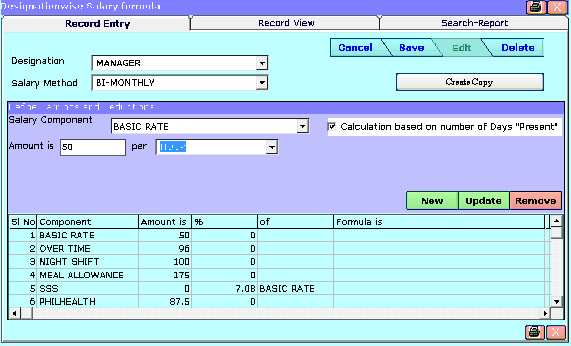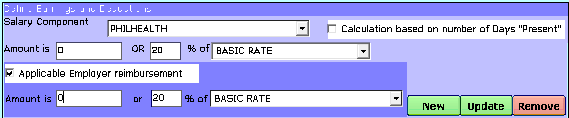Designation wise Salary Formula Previous Top Next

Designation wise Salary Formula:
This module is to create formulas for salary calculation. For each designation salary method wise formulas to be defined.
Create Copy : This button is to create a copy of rule for another designation with predefined clause of selected designation.Salary Component : Which is in the list where already defined in salary component module.
Calculation based on number of Days "Present": option is for consider the present+paid leaves to Component wise salary calculation.
here will get options are changing as per rules defined per salary component.

Example 1:
Bellow example shown Meal allowance is calculated base on 10% of Basic Rate.Example 2:
Bellow example shown Philhealth is calculated base on 20% of Basic Rate. This salary code is also consider to be employer contribution. Hence here defined Employer Contribution is 20% of Basic rate.